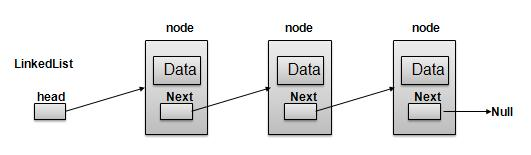{{ message }}

Instantly share code, notes, and snippets.

# Thuy ThuyNT13

Last active Mar 30, 2022
Big O Notation
View Big_O_Notation.md

Big O notation is a way for us to describe how long it takes for an algorithm to run. We can use Big O notation to compare how efficient different approaches to solving a problem are. In big O notation we describe the runtime of an algorithm in terms of how quickly the runtime grows as the input to the algorithm gets very, very large. Let’s break down the definition a bit:

• How quickly the runtime grows:

• We can’t give an exact amount of time that an algorithm will run for because it depends on too many things. Is it running on a supercomputer, a really old desktop, or a calculator? Which language is it written in? Is the computer running anything else at the same time? All of these can affect the runtime, so we remove these variables by focusing on how the runtime grows.
• Based on the input:

• Since we are removing runtime from the description we need another way to express the speed - we can’t use seconds anymore. Instead we’ll use the size of the input to describe it. We will use

Last active Jan 15, 2018

Linked lists are a way to store sequential data. A linked list is made up of one or more list nodes that contain the data and a link or pointer to the next node in the sequence. We call the start of the linked list the head and the end of the linked list the tail.### Why would we use a linked list?

• Constant time insertions/deletions
• Easily expandable
Last active Jun 1, 2018
Thùy Ngô resume
View Thuy_Ngo-resume.md

# Thùy T. Ngô

(562) 308-8091  |  thuy@thuy.life  |  Website  |  GitHub  |  LinkedIn

## SKILLS & TOOLS

Languages:   Ruby, JavaScript, Java, SQL, HTML, CSS, Bash

Last active Nov 5, 2018
implement a Rolling Hash in C#
View RollingHash.cs
This file contains bidirectional Unicode text that may be interpreted or compiled differently than what appears below. To review, open the file in an editor that reveals hidden Unicode characters. Learn more about bidirectional Unicode characters
 /* First calculate the hash of first *n* letter substring (abcd) in string. Iterate through string, starting at index of next (= pattern length): subString = (first + subSubstring) + next 1. drop a (first) (a*7^0 + b*7^1 + c*7^2 + d*7^3) - a*7^0 2. divide everything by 7 (b*7^1 + c*7^2 + d*7^3) / 7 => b*7^0 + c*7^1 + d*7^2